## Fractions 2

PIN number: SWGAOM

1.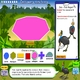### L86 Cassowary sanctuary

• Learning area Mathematics, Science
• Published 06/03/2018
• TLF-ID L86

Help a park ranger to arrange fencing in a wildlife sanctuary. Divide common geometric shapes into equal-sized sections for keeping cassowaries. Group the enclosures to form a quarantine zone for sick and injured birds. Then express divisions of the enclosures as fractions. Work through facts about the life of cassowaries: physical characteristics; diet; habitat; life cycles; and locations. Interact with graphs to see how people can help to save cassowaries. Answer questions as you go; express your answers as fractions. This learning object is a combination of two objects in the same series.

2.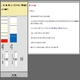### L3521 Fractions: comparing

• Learning area Mathematics
• Published 19/05/2016
• TLF-ID L3521

Use visual representations of two fractions to find a common denominator. Write the two fractions as equivalences with the common denominator. Place the fractions on a number line. Find new fractions between the two original fractions. This learning object is one in a series of seven objects. This resource requires the current Java Plug-in to be installed.

3.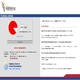### L3523 Fractions: naming

• Learning area Mathematics
• Published 30/05/2016
• TLF-ID L3523

Name a fraction that is represented visually. This object is one in a series of seven objects. This resource requires the current Java Plug-in to be installed.

4.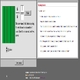### L3651 Fractions: equivalent

• Learning area Mathematics
• Published 30/05/2016
• TLF-ID L3651

Manipulate a visual representation of a fraction to find and name an equivalent fraction. This object is one in a series of seven objects. This resource requires the current Java Plug-in to be installed.

5.### L125 Neighbourhood fractions

• Learning area Mathematics
• Published 31/03/2016
• TLF-ID L125

Help a town planner to design site plans. Assign regions on a 10x20 grid for different uses. Calculate the percentage and the fraction of the total site used for each region. Use a number line to display fractions and equivalent decimals. Design site plans for a: 1. Neighbourhood; 2. City; 3. Farm. This learning object is a combination of three objects in a series.

6.### L134 Dynamic fractions

• Learning area Mathematics
• Published 31/03/2016
• TLF-ID L134

Use a tool to explore how to express fractions and display them in different ways. Create a grid with a number of rectangles ranging from 1 to 100 (up to a 10x10 array). Select boxes within the grid and view or enter corresponding fractions and their equivalents. Interact with a dynamic number line to express fractions differently. Change display options to set task difficulty. Make your own question and answer games.

7.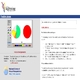### L3520 Fraction pieces

• Learning area Mathematics
• Published 19/05/2016
• TLF-ID L3520

Place fractions of a circle or square on a whole. Investigate equivalence. This learning object is one in a series of seven objects. This resource requires the current Java Plug-in to be installed.

8.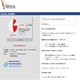### L3526 Fractions: visualising

• Learning area Mathematics
• Published 30/05/2016
• TLF-ID L3526

Manipulate a rectangle or circle to represent given fractions. This object is one of a series of seven objects. This resource requires the current Java Plug-in to be installed.

9.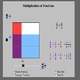### L3525 Fractions: rectangle multiplication

• Learning area Mathematics
• Published 30/05/2016
• TLF-ID L3525

Explore an interactive model that represents the product of two fractions including proper and improper fractions. Use the model to find the product of a given set of fractions including proper and improper fractions. For example, 1/3 x 5/9 = 5/27 and 4/3 x 2/5 = 8/15. This object is one of a series of seven objects. This resource requires the current Java Plug-in to be installed.

10.### L6542 Exploring fractions

• Learning area Mathematics
• Published 15/03/2016
• TLF-ID L6542

Use partially filled measuring cups to explore fractions: improper, mixed and equivalent fractions. For example, select six cups which are one-quarter full to balance one and a half cups. Or achieve an equivalent result by selecting three cups, which are half full.

11.### L2800 Fraction fiddle: tool

• Learning area Mathematics
• Published 31/03/2016
• TLF-ID L2800

Use a tool to explore what happens when you change the numerals in a fraction. Compare two fractions and find out which is larger. For example, decide whether 4/3 is larger than 7/5. Build the two fractions. Compare the fractions on a number line. A handy tool for use on an interactive whiteboard.

12.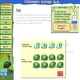### L119 Cassowary ecology quiz

• Learning area Mathematics, Science
• Published 06/03/2018
• TLF-ID L119

Explore facts about the life of cassowaries: physical characteristics; diet; habitat; life cycles; and locations. Interact with graphs to see how much people can help cassowaries. Work through ecology notes and resources. Answer questions as you go; express your answers as fractions. This learning object is one in a series of two objects.